# What are d-block metals?

| Home | | Inorganic Pharmaceutical Chemistry |

## Chapter: Essentials of Inorganic Chemistry : Transition Metals and d-Block Meta Chemistry

The elements in groups 3–12 as shown in the schematic periodic table below are defined as the so-called d-block metals. The term transition metal is also often used to describe this group of elements.

What are d-block metals?

The elements in groups 3–12 as shown in the schematic periodic table below are defined as the so-called d-block metals. The term transition metal is also often used to describe this group of elements. However, the IUPAC (International Union of Pure and Applied Chemistry) defines transition metals as elements with an incomplete d subshell or elements that can form a cation with an incomplete d subshell. Therefore, the group 12 metals zinc (Zn), cadmium (Cd) and mercury (Hg) are not typically classified as transition metals (Figure 7.1) .Elements in the f-block, the so-called lanthanides and actinides, have been in the past called inner transition metals. Nowadays, they are more often referred to as f-block elements and selected examples will be discussed in Chapter 11.

Each group of d-block metals is formed by three members and is therefore called a triad. Sometimes, elements are grouped according to their chemical behaviour. One example is the group of platinum group metals, which encompasses ruthenium (Ru), osmium (Os), palladium (Pd) and platinum (Pt). Sometimes, you can find the term heavier d-block metals, which refers to d-block metals of the second and third row.

## Electronic configurations

Generally, the ground-state electronic configurations of the first, second and third-row d-block metals follows the progressive filling of the 3d, 4d and 5d atomic orbitals, respectively. Nevertheless, there are exceptions, such as the ground state of chromium, which is [Ar]4s13d5 rather than [Ar]4s23d4. The reasons are fairly complicated and will not be further discussed in this book (Table 7.1).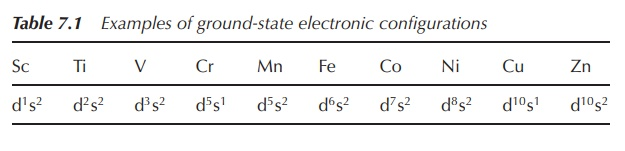d-Block metals can show several oxidation states as their valence electrons can be present in more than one atomic orbital. M2+ and M3+ ions of the first-row d-block metals follow the general formula [Ar]3dn. The electronic configurations for second- and third-row d-block metals are again more complicated and will not be further discussed in this book.

## Characteristic properties

Nearly all d-block metals are hard, malleable and ductile, and conduct electricity and heat. Most of them will form one of the typical metal structures. Exceptions are manganese (Mn), Zn, Cd and Hg. Only three d-block elements, namely iron (Fe), cobalt (Co) and nickel (Ni), are known to produce a magnetic field.

d-Block metals tend to readily form complexes with a characteristic colour if their ground-state electronic configuration is different from d0 or d10. Complex formation is often characterised by a colour change. For example, [CoF6]3− is green, whereas [Co(NH3)H2O]3+ is red and [Co(H2O)6]3+ is blue.

Coordination complexes are defined as chemical structures that consist of a central atom or metal ion, and the surrounding molecules or anions called the ligands.

Paramagnetism is a phenomenon that is often observed for d-block metal compounds. This is a result of the presence of unpaired electrons and can be investigated using electron paramagnetic resonance (EPR) spectroscopy. As a result, abnormalities in the NMR spectra can be observed such as broadening of the signals or unusual chemical shifts.

Paramagnetism is defined as the phenomenon whereby some materials show magnetic properties only once they are exposed to a magnetic field. Outside this magnetic field, no magnetic properties are seen. This is in contrast to ferromagnets, which show magnetic properties independent of the environment.

## Coordination numbers and geometries

d-Block metal compounds readily form complexes displaying different coordination numbers and geome-tries. In this section, we will restrict our discussion to complexes with only one metal centre (mononuclear complex). Also, it is important to note that the discussed geometries are regular geometries, which in practice can often be distorted as a result of steric hindrances. Additionally, in reality, fluxional behaviour in solution can be observed if the energy difference between the different structures is small enough, but we will restrict the following discussion to the solid state of complexes.

In general, steric and electronic factors dictate the coordination number. Sterically demanding ligands are more likely to form complexes with a low coordination number. In contrast, complexes containing small ligands and a large metal centre favour high coordination numbers (Figure 7.2).Figure 7.2 Common geometries of metal complexes . (a) Linear, (b) trigonal, (c) tetrahedran, (d) square planar, (e) trigonal bipyramid, (f) square pyramid, (g) octahedron, (h) pentagonal bipyramid and (i) tricapped trigonal prism (Reproduced with permission from . Copyright © 2009, John Wiley & Sons, Ltd.)

The Kepert model is typically used to describe the shape of d-block metal complexes. The metal is defined to be the centre of the complex, and the ligands are arranged freely on a sphere around the centre. Only ligands are taken into consideration when determining the geometry of the complex. This is in contrast to the valence shell electron pair repulsion (VSEPR) model, which is used to determine the structure of p-block element compounds, where also nonbonding electrons are considered.

The η-nomenclature for ligands is used in organometallic chemistry and describes the number of atoms in a ligand that directly interact with the metal. The prefix η (eta) is accompanied by a number, which equals the number of atoms coordinating to the metal. This is called hapticity of a ligand.

### 1. Coordination number 2: linear

A coordination number of 2 can be typically found for the metals Cu(I),1 Ag(I), Au(I) and Hg(II). The metal forms the centre of the complex, and the two ligands are arranged at 180 to each other (Figure 7.3).### 2. Coordination number 3: trigonal planar or trigonal pyramidal (less common arrangement)

In general, trifold coordination of a metal centre is not very common. There are examples of metals with a full d-orbital (d10 metals), which form trigonal planar structures. This means three ligands are arranged around the metal centre in one plane with 120 angle to each other. Examples include complexes of Cu(I), for example, in [Cu(CN)3]2− and Ag(I), Au(I), Hg(II) and Pt(0) in [Pt(PPh3)3] (Figure 7.4).### 3. Coordination number 4: tetrahedral or square planar

The coordination number 4 is extremely common for d-block metal complexes. Most frequently, a tetrahedral arrangement can be observed. Examples include [MnO4]2−, [FeCl4] and [CrO4]2− (Figure 7.5).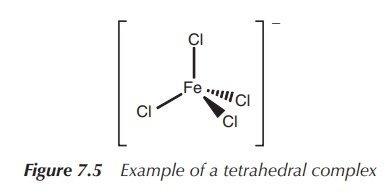The square planar arrangement in which all four ligands are arranged around the metal centre in one plane is less commonly observed and often connected to d8 metals. Nevertheless, some of these complexes are very important as a result of their medical application. For example, the square planar complex [PtCl4]2− is commonly used as a precursor to the known chemotherapeutic agent cisplatin, whereas square planar complexes [PdCl4]2−, [AuCl4] and [RhCl(PPh3)3] are all under investigation for their use in medicine (Figure 7.6).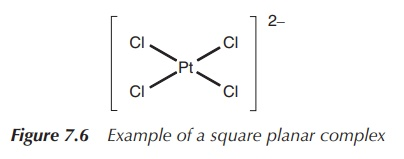### 4. Coordination number 5: trigonal bipyramidal or square-based pyramidal

The energy difference between the trigonal bipyramidal structure and the square-based pyramidal structure is usually fairly small and therefore many structures lie in reality between those two. Examples for simple trigonal bipyramidal structures include [CdCl5]3− and [CuCl5]3−, whereas [WCl(O)] and [TcCl4(N)] form square-based pyramidal structures typical for a series of oxo and nitrido complexes (Figure 7.7).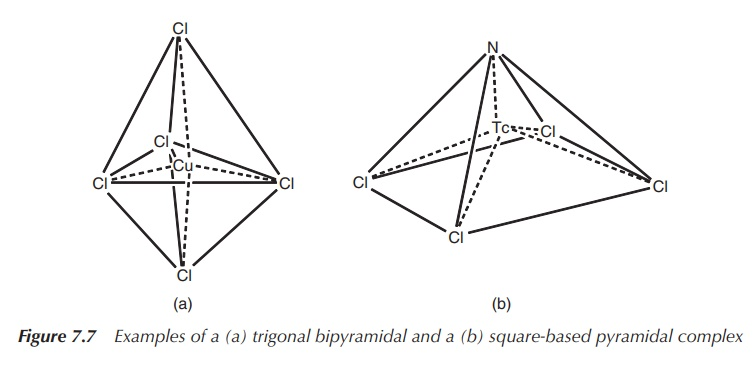### 5. Coordination number 6: octahedral or trigonal prismatic (less common geometry)

Octahedral geometry is most commonly observed for the coordination number 6. Metals of all kinds of elec-tronic configurations form octahedral complexes, for example, [Mn(OH2)6]3+, [V(OH2)6]3+, [Fe(CN)6]3− and [Fe(OH2)6]2+ (Figure 7.8).It was long believed the octahedral geometry is the only geometry for coordination number 6 in existence, but eventually examples of trigonal prismatic coordination have been confirmed by X-ray analysis. Examples are [ZrMe6]2− and [ReMe6] (Figure 7.9).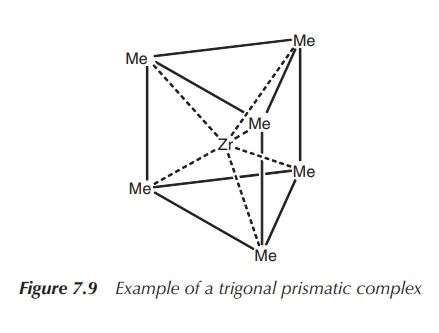### 6. Coordination number 7 and higher

Coordination numbers of 7 and higher are most commonly observed for d-block metals of the second and third row. Geometries can become fairly complicated and are not further discussed here.

## Crystal field theory

Many transition-metal complexes are coloured. This and other spectroscopic properties, such as magnetism and hydration enthalpies, can be explained with the so-called crystal field theory (CFT).

The CFT describes the degeneration of the d- and f-orbitals in transition-metal complexes. It does not attempt to describe any type of chemical bonds.

CFT is based on the interaction of a positively charged cation and the nonbinding (negatively charged) electrons of the ligand. The general principle is that the five d orbitals are degenerated, meaning that they do not occupy the same energy level anymore. Once the ligands approach the central positively charged cation, the electrons of the ligands will become closer to some of the d orbitals of the metal. This results in the degeneration of the d orbitals. Electrons in d orbitals that are closer to the ligands will occupy a higher energy level as the negatively charged electrons will repel each other (Figure 7.10).The most common type of transition-metal complexes is octahedral complexes, where the central metal is coordinated by six ligands. The five d orbitals are split into two sets with the same energy level, the energy difference is called Δoct. The orbitals dxy, dxz and dyz occupy the lower energy level as they are further away from the ligands. Ligands can be seen as approaching the central metal along the axes, where the orbitals dxy, dxz and dyz, referred to as t2g, are located in between the axes. In contrast, the two remaining d orbitals dx2y2 and dz2 , referred to as eg, are positioned along the axes (from where the ligands approach) and there-fore occupy a higher energy level within an octahedral complex.

Figure 7.11 shows how energy levels of d orbitals change, ranging from the energy of d orbitals of the free metal ion, followed by the energy of d orbitals of the complex in a spherical field and the split energy levels observed in an octahedral field.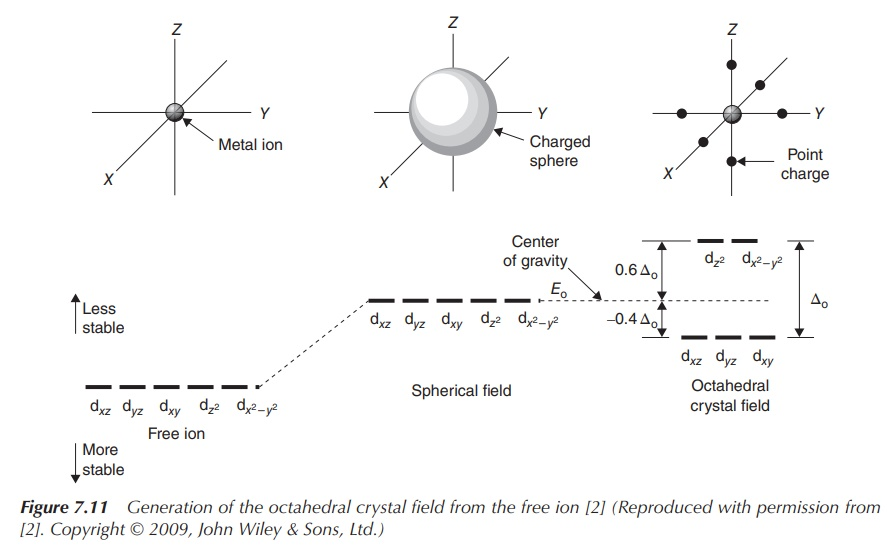There are several factors influencing this splitting of the d orbitals:

• The metal ion itself.

• The oxidation state of the metal ion: The energy difference  increases with increasing oxidation state for any given metal.

• The nature of the ligand: Some ligands encourage a large value for , whilst the formation of complexes with other ligands results in a small splitting of t2g and eg orbital sets. The spectrochemical series is a list that shows ligands in the order in which they produce a splitting, ranging from small to large Δ:

I < Br < S2− < SCN < Cl < NO3 < N3 < F < OH < C2O42− < NH3 < NO2 < PPh3 < CN < CO

• The geometry of the transition-metal complex: The arrangement of energy level changes and is dependent on the geometry of the complex.Figure 7.11 shows the energy diagram for octahedral complexes. In Figure 7.12, the CFT splitting diagrams for other geometries, including tetrahedral, square planar and trigonal bipyramidal complexes are shown.

It is interesting to observe what happens when these orbitals are filled with electrons. The lowest energy levels will be filled first, and Hund’s rule states that orbitals are filled with one electron first, before the elec-trons are paired. The Pauli Exclusion Principle states that two electrons in the same orbital are not allowed to have the same spin; nevertheless, it consumes energy to change the spin of an electron. Looking at octahedral complexes and the energy scheme shown in Figure 7.11, filling the orbitals with one, two or three electrons is straightforward. Each of the t2g orbitals will be filled with one electron. The addition of the next electron leads now to two possibilities. Electron number 4 can either be added to a vacant eg orbital, which means the energy ΔOct is needed to promote it to this level. These types of complexes are called high-spin complexes. Alternatively, the fourth electron can be added to one of the already occupied orbitals of t2g once the electron spin has changed. These complexes are called as low-spin complexes. Depending on the size of ΔOct, different types of complex are formed. If ΔOct is very large, it consumes less energy to fill a t2g orbital with a second electron; vice versa, if ΔOct is relatively small, it is energetically favourable to promote the fourth electron to the eg level.

Examples of a low- and high-spin complex with four electrons in the d orbitals are shown in Figure 7.13. Both complexes have the same metal ion in the same oxidation state as the centre, and ΔOct depends only on the ligands. The same discussion follows for the addition of a fifth electron.As mentioned at the beginning of this chapter, CFT can be used to explain the colour of these often brightly coloured transition metals. When a molecule absorbs a photon, one or more electrons are temporarily pro-moted within the set of split d orbitals from the lower to the higher energy level. This leads to a complex in an excited state, and the energy difference to the ground state equals the energy of the absorbed photon. The latter energy is inversely related to the wavelength of the light absorbed. Therefore the transition-metal complex can be seen in the complementary colour.

Contact Us, Privacy Policy, Terms and Compliant, DMCA Policy and Compliant

TH 2019 - 2022 pharmacy180.com; Developed by Therithal info.